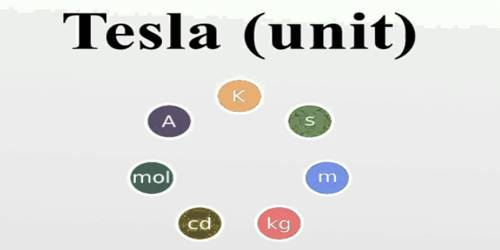# One Tesla

One Tesla: If 1 coulomb of charge is in the motion perpendicular to the direction of magnetic field with a velocity of 1 ms-1 and experiences a force of 1 N, the magnitude of that magnetic field is called 1 testa (T).

The Tesla (symbolized T) is the usual unit of magnetic flux density. It is the same to one weber per meter squared (1 Wb · m-2). Reduced to base units in the International System of Units (SI), 1 T represents one kilogram per second squared per ampere (kg · s-2 · A-1). The Tesla was named for Nikola Tesla, the Serbian-American scientist who contributed significantly headed for the improvement of modern-day electrical power systems.

A particle, carrying a charge of one coulomb, and moving perpendicularly through a magnetic field of one tesla, at a speed of one metre per second, experiences a force with magnitude one newton, according to the Lorentz force law. As an SI derived unit, the tesla can also be expressed as-

T = V.s / m2 = N / A.m = J / A.m2 = H.A / m2 = kg / C.s = N.s / C.m = kg / A.s2

Units used:

A = ampere, C = coulomb, kg = kilogram, m = metre, N = newton, s = second, H = henry, V = volt, J = joule and Wb = weber.